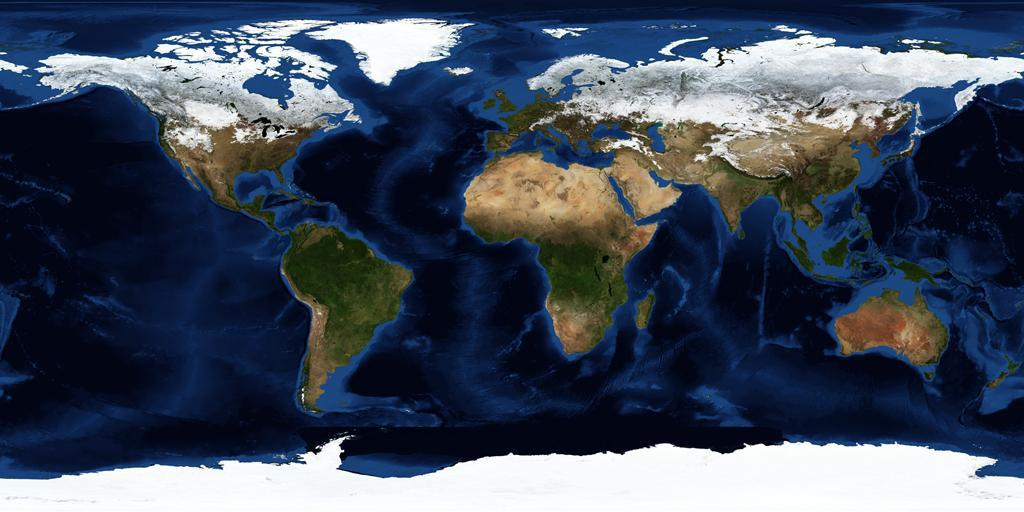Source Projections

The following projections can be used as the source for a conversion to the TOAST format:

# Equirectangular Projection🔗

With Equirectangular coordinates, every pixel represents the same number of degrees of latitude and longitude for planets, and degrees for Right Ascension (RA) and Declination (Dec) for sky surveys. Equirectangular projection is by far the most common system used to represent earth maps, such as the NASA Blue Marble map shown below. The utility of this projection is greatly assisted by the simple fact that very few people live near the poles, where the projection becomes problematic, in particular all the points along the top of an equirectangular projection represent only one point in 3D space, namely the North Pole. And of course there is a similar singularity for the lower edge of the map and the South Pole.This composite image of Earth was taken in January 2004. (Credit: NASA, Visible Earth.)

The wider problem with equirectangular projections is that the relative sizes of objects (land masses in the map above) are substantially incorrect. An equirectangular image that covers the entire sphere will have a 2×1 aspect ratio, so it is two times as wide as it is high (a full circumference east-west, half a circumference north-south).

# Mercator Projection🔗

Unlike equirectangular projection, Mercator projection preserves angles, so is useful in marine navigation, but at the expense of distorting the relative sizes of land masses. The distortion of sizes is even greater than in equirectangular projection, with Greenland appearing about the same size as Africa in Mercator projection, about half the size in equirectangular projection, and about one fourteenth the size on a globe.

Mercator projection is a specific example of cylindrical projection — where horizontal lines are preserved in the mapping.

# Gnomonic Projection🔗

A flat map is achieved by projecting from the center of the sphere, perpendicularly through the surface of the sphere, to a tangent plane. Unlike the previous two projection systems gnomonic only enables less than half of the sphere to be projected onto one map. Also known as tangential projection, gnomonic projection displays all great circles as straight lines, so for example, the meridians and equator appear as straight lines on the map.